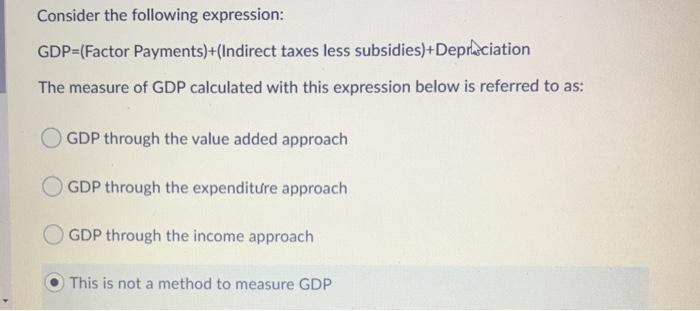### Create an Account

Home / Questions / Consider the following expression: GDP=(Factor Payments)+(Indirect taxes less subsidies)+D...

# Consider the following expression: GDP=(Factor Payments)+(Indirect taxes less subsidies)+Depræciation The measure of GDP calculated with this expression below is referred to as: GDP through the value

Consider the following expression: GDP=(Factor Payments)+(Indirect taxes less subsidies)+Depræciation The measure of GDP calculated with this expression below is referred to as: GDP through the value added approach OGDP through the expenditure approach GDP through the income approach This is not a method to measure GDPMay 07 2021 View more View LessSubscribe To Get Solution